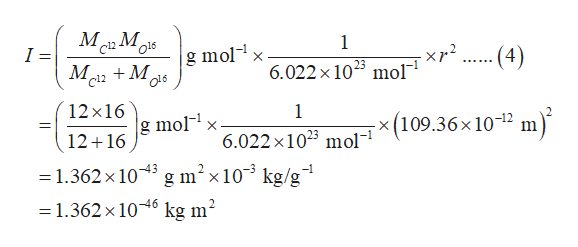# Calculate the frequency of the J = 7 ← 6 transition in the pure rotational spectrum of 12C16O. Assume the equilibrium bond length is 109.36pm.Frequency is _____ HWhat is the corresponding wavenumber? ______ cm-1

Question
70 views

Calculate the frequency of the J = 7 ← 6 transition in the pure rotational spectrum of 12C16O. Assume the equilibrium bond length is 109.36pm.

Frequency is _____ H

What is the corresponding wavenumber? ______ cm-1

check_circle

Step 1

The energy in the term of corresponding wavenumber for the rotational region spectra (ƐJ) is calculated as show in equation (1) where h and c is the Planck’s constant and speed of light respectively, I is the moment of inertia, and J is the rotational energy level.

Step 2

The emitted energy in the term of wavenumber is calculated as shown in equation (2). This will give the equation (3).

Step 3

The moment of inertia for the given molecule is calculated as shown in equation (4) where MC12 and MO16 are molar masses of carbon and oxygen, and r is the bond length. The values for r, MC12, and MO16 are substituted...help_outlineImage TranscriptioncloseМ М, c12 1 o16 I = g molx -- (4) - xp2 M2M 6.022 x 1023 mol 12x16 1 g molx - x (109.36x 10-12 m 12 16 6.022 x 1023 mol g m2x10 kg/g = 1.362x 103 = 1.362x106 kg m2 fullscreen

### Want to see the full answer?

See Solution

#### Want to see this answer and more?

Solutions are written by subject experts who are available 24/7. Questions are typically answered within 1 hour.*

See Solution
*Response times may vary by subject and question.
Tagged in

### Chemistry